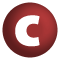# DynamicVariable

#### class DynamicVariable[T] extends AnyRef

DynamicVariables provide a binding mechanism where the current value is found through dynamic scope, but where access to the variable itself is resolved through static scope.

The current value can be retrieved with the `value` method. New values should be pushed using the `withValue` method. Values pushed via `withValue` only stay valid while the `withValue`'s second argument, a parameterless closure, executes. When the second argument finishes, the variable reverts to the previous value.

Usage of `withValue` looks like this:

``` someDynamicVariable.withValue(newValue) {
// ... code called in here that calls value ...
// ... will be given back the newValue ...
}
```

Each thread gets its own stack of bindings. When a new thread is created, the DynamicVariable gets a copy of the stack of bindings from the parent thread, and from then on the bindings for the new thread are independent of those for the original thread.

1. AnyRef
2. Any

### Value Members

1. #### def equals(arg0: Any): Boolean

This method is used to compare the receiver object (`this`) with the argument object (`arg0`) for equivalence

This method is used to compare the receiver object (`this`) with the argument object (`arg0`) for equivalence.

The default implementations of this method is an equivalence relation:

• It is reflexive: for any instance `x` of type `Any`, `x.equals(x)` should return `true`.
• It is symmetric: for any instances `x` and `y` of type `Any`, `x.equals(y)` should return `true` if and only if `y.equals(x)` returns `true`.
• It is transitive: for any instances `x`, `y`, and `z` of type `AnyRef` if `x.equals(y)` returns `true` and `y.equals(z)` returns `true`, then `x.equals(z)` should return `true`.

If you override this method, you should verify that your implementation remains an equivalence relation. Additionally, when overriding this method it is often necessary to override `hashCode` to ensure that objects that are "equal" (`o1.equals(o2)` returns `true`) hash to the same `Int` (`o1.hashCode.equals(o2.hashCode)`).

arg0

the object to compare against this object for equality.

returns

`true` if the receiver object is equivalent to the argument; `false` otherwise.

definition classes: AnyRef ⇐ Any
2. #### def hashCode(): Int

Returns a hash code value for the object

Returns a hash code value for the object.

The default hashing algorithm is platform dependent.

Note that it is allowed for two objects to have identical hash codes (`o1.hashCode.equals(o2.hashCode)`) yet not be equal (`o1.equals(o2)` returns `false`). A degenerate implementation could always return `0`. However, it is required that if two objects are equal (`o1.equals(o2)` returns `true`) that they have identical hash codes (`o1.hashCode.equals(o2.hashCode)`). Therefore, when overriding this method, be sure to verify that the behavior is consistent with the `equals` method.

definition classes: AnyRef ⇐ Any
3. #### def toString(): String

Returns a string representation of the object

Returns a string representation of the object.

The default representation is platform dependent.

4. #### def value: T

Retrieve the current value

Retrieve the current value

5. #### def value_=(newval: T): Unit

Change the currently bound value, discarding the old value

Change the currently bound value, discarding the old value. Usually `withValue()` gives better semantics.

6. #### def withValue[S](newval: T)(thunk: ⇒ S): S

Set the value of the variable while executing the specified thunk

Set the value of the variable while executing the specified thunk.

newval

The value to which to set the variable

thunk

The code to evaluate under the new setting